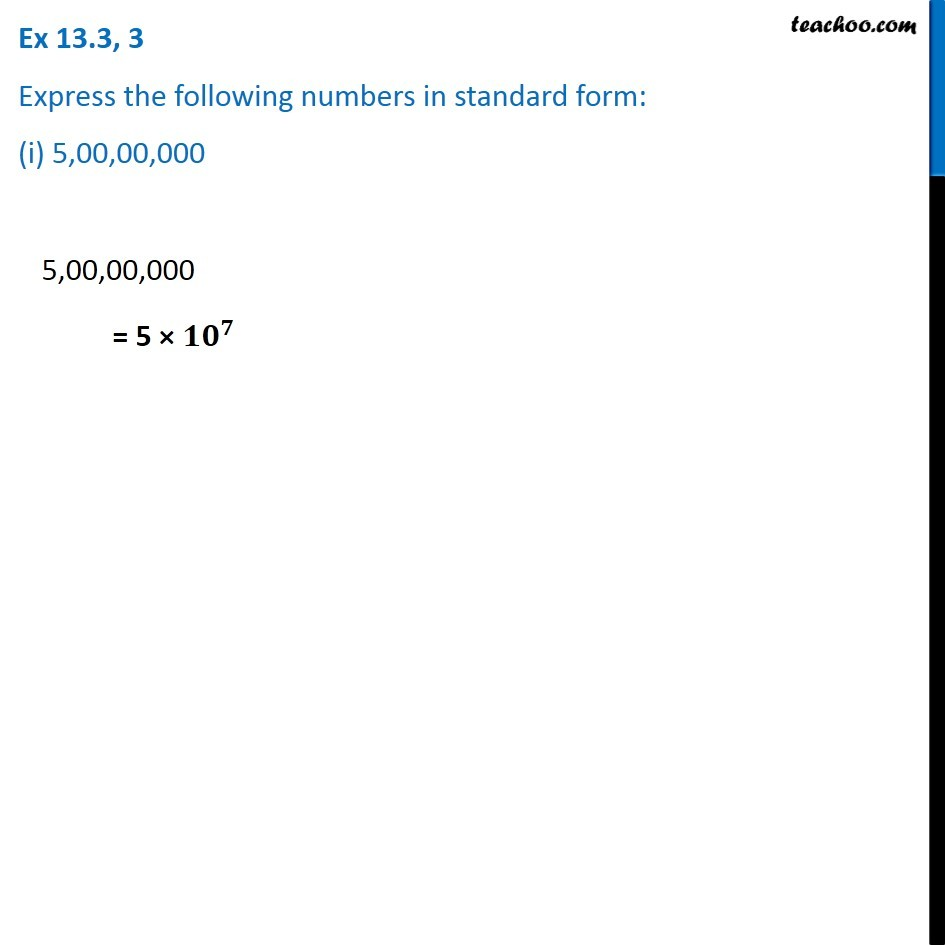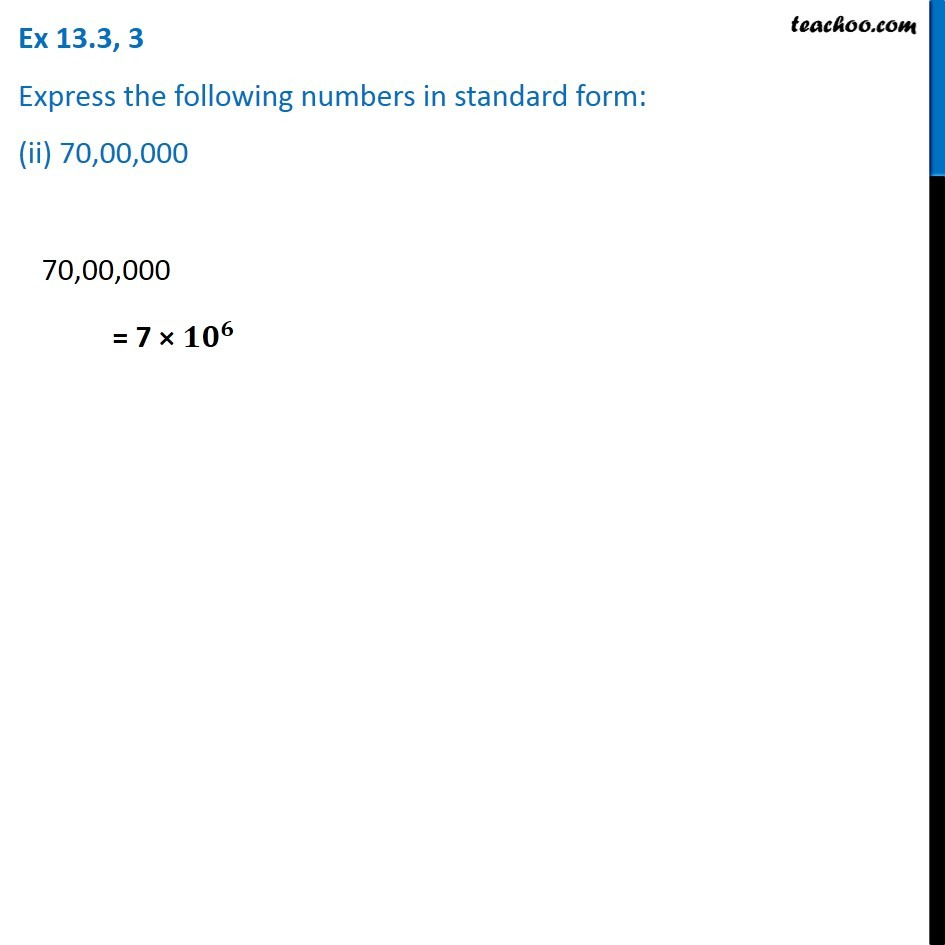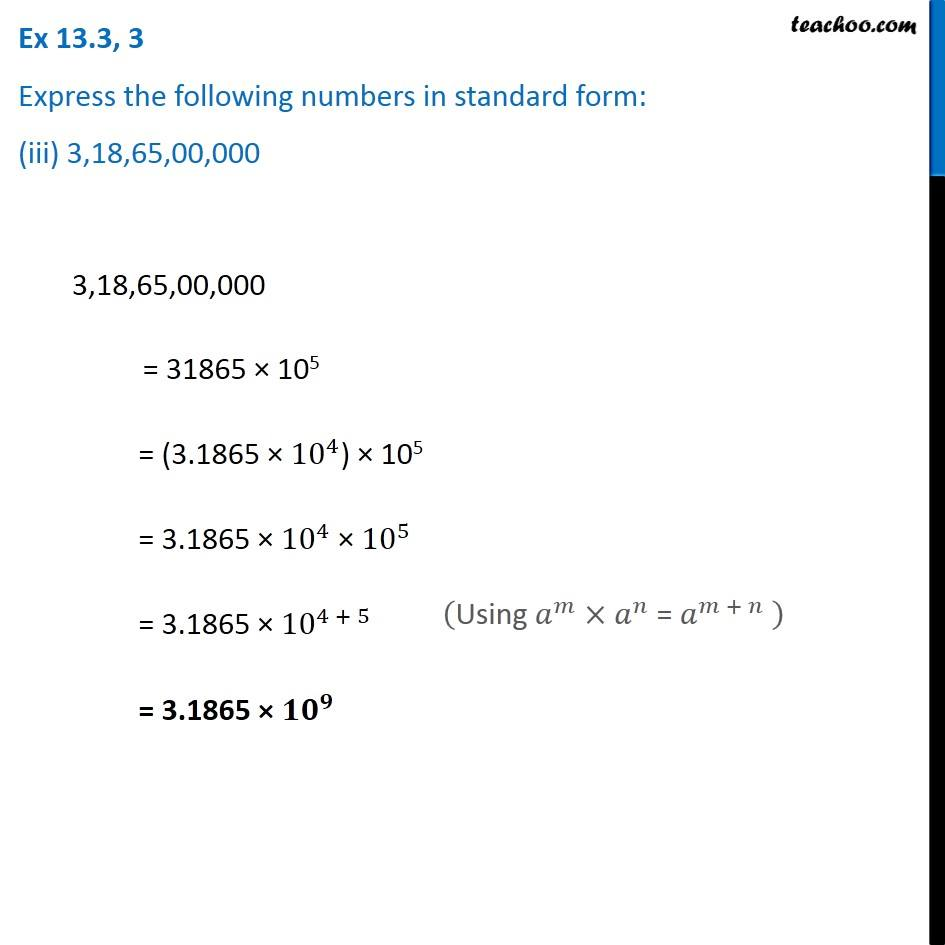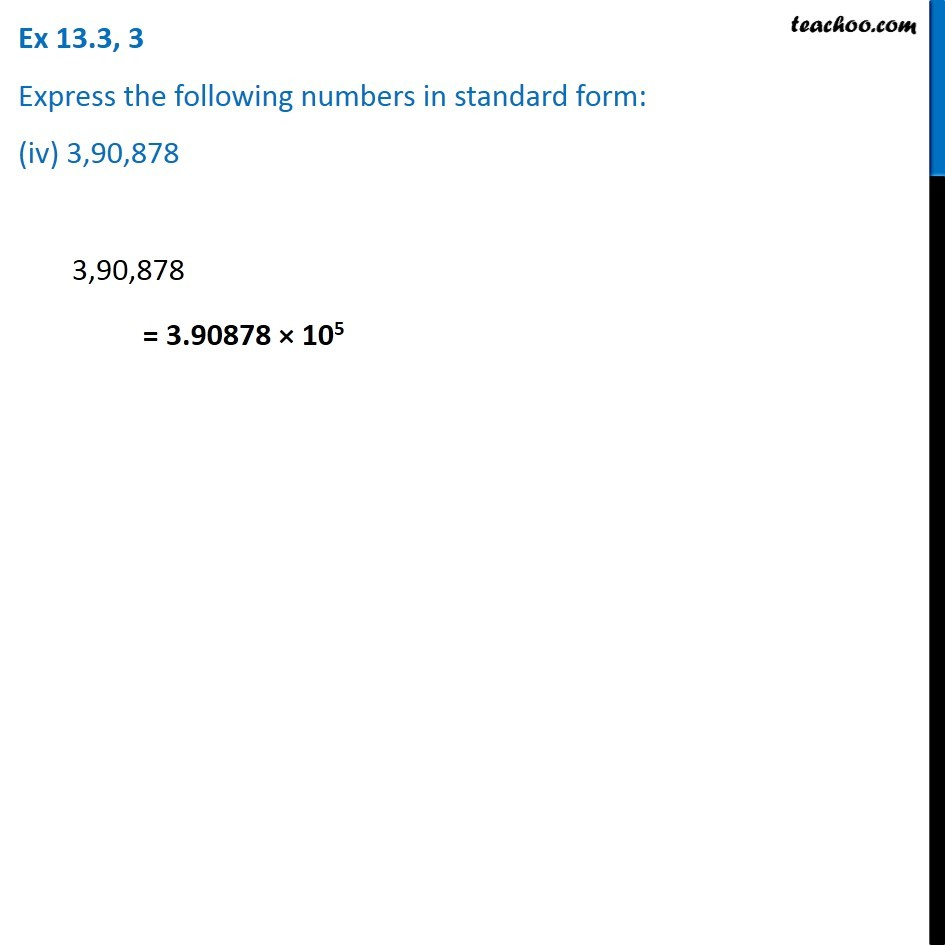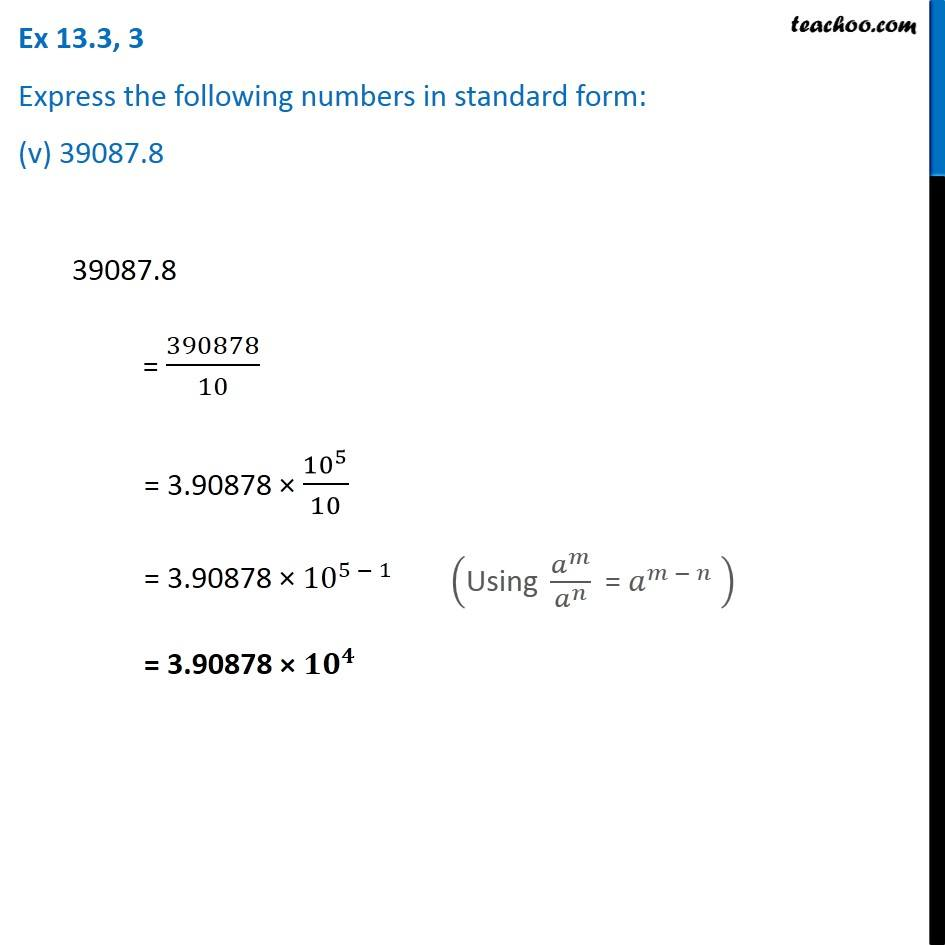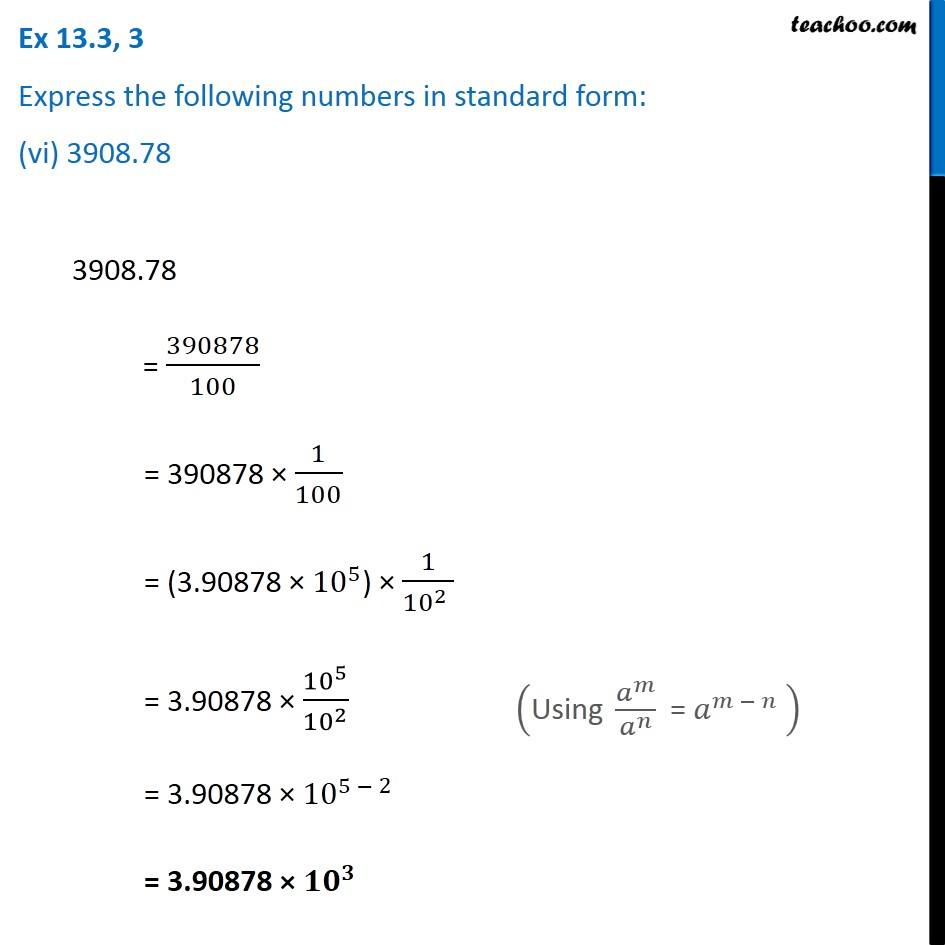Subscribe to our Youtube Channel - https://you.tube/teachoo

1. Chapter 13 Class 7 Exponents and Powers
2. Serial order wise
3. Ex 13.3

Transcript

Ex 13.3, 3 Express the following numbers in standard form: (i) 5,00,00,000 5,00,00,000 = 5 × 〖𝟏𝟎〗^𝟕 Ex 13.3, 3 Express the following numbers in standard form: (ii) 70,00,000 70,00,000 = 7 × 〖𝟏𝟎〗^𝟔 Ex 13.3, 3 Express the following numbers in standard form: (iii) 3,18,65,00,000 3,18,65,00,000 = 31865 × 105 = (3.1865 × 〖10〗^4) × 105 = 3.1865 × 〖10〗^4 × 〖10〗^5 = 3.1865 × 〖10〗^(4 + 5) = 3.1865 × 〖𝟏𝟎〗^𝟗 ("Using " 𝑎^𝑚×𝑎^𝑛 " = " 𝑎^(𝑚 + 𝑛) " " ) Ex 13.3, 3 Express the following numbers in standard form: (iv) 3,90,878 3,90,878 = 3.90878 × 105 Ex 13.3, 3 Express the following numbers in standard form: (v) 39087.8 39087.8 = 390878/10 = 3.90878 × 〖10〗^5/10 = 3.90878 × 〖10〗^(5 − 1) = 3.90878 × 〖𝟏𝟎〗^𝟒 ("Using " 𝑎^𝑚/𝑎^𝑛 " = " 𝑎^(𝑚 − 𝑛) " " ) Ex 13.3, 3 Express the following numbers in standard form: (vi) 3908.78 3908.78 = 390878/100 = 390878 × 1/100 = (3.90878 × 〖10〗^5) × 1/(〖10〗^2 ) = 3.90878 × 〖10〗^5/〖10〗^2 = 3.90878 × 〖10〗^(5 − 2) = 3.90878 × 〖𝟏𝟎〗^𝟑 ("Using " 𝑎^𝑚/𝑎^𝑛 " = " 𝑎^(𝑚 − 𝑛) " " )

Ex 13.3